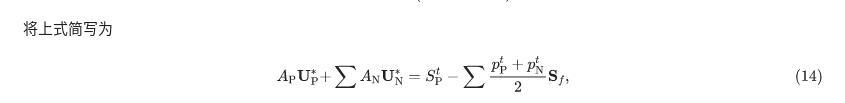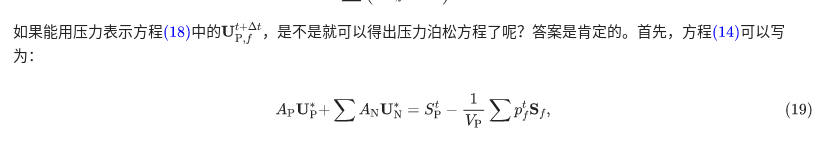# Re: icoFoam解析中的问题

• @warnerchang

26式从里往外：$p^{t+\Delta t}$（标量）

1. $p^{t+\Delta t}_f$（表面力在面上的梯度，矢量，在面法向的投影）

2. $\sum p^{t+\Delta t}_f \mathbf{S}_f$（矢量的面积分，散度，标量）

3. $(\frac{1}{V_p}\sum p^{t+\Delta t}_f \mathbf{S}_f)_f$（标量在面上的梯度，矢量）

4. $\sum\frac{1}{A_{P,f}}(\frac{1}{V_p}\sum p^{t+\Delta t}_f \mathbf{S}_f)_f\cdot\mathbf{S}_f$（矢量的面积分，散度，标量）

你刚开始问的先求散度再求梯度指的是2、3。

为什么把加下标 $_f$ 称为梯度操作，这里虽然没有矢量，却人为规定了p是面上力垂直于面的分量（正应力），面上力平行于面的分量放到切应力里了。所以 $p_f$ 就是压力的梯度在面法向上的投影，它乘以面矢量 $\mathbf{S}_f$ 再求和就是计算散度。（这里准确来说不是梯度。如果对一个标量场求偏导数，通常称最大偏导数为梯度，最大偏导数的增长方向为梯度方向。这个可以说是梯度在面法向上的投影。即（7）式上面一行提到的“面法向梯度”。）

如果要理解最好给这个通过面积分计算散度的过程取个名字，比如叫“封闭面积分”
，因为“散度”更像是一个物理量而不是一个操作过程。散度就像质量、转动惯量一样是个客观属性，封闭面积分 是计算散度的一种方法，另一种方法是直接对散度进行体积分。两种方法结果相等叫高斯定理。

散度的值就是封闭体内“源”的“数量”。如果换成电比较好理解。散度就是封闭体内有几个电荷。梯度就是两点间电荷量差值（电压）（$电压=\frac{电荷量}{电容}$），当然是个方向中差值最大的那个。

这样，梯度是用电荷量(标量)求电场（有向电压），散度是通过电场（有向电压）对面积分求封闭体内电荷（相对的）。这两个操作是互逆的，区别点在，经过梯度、散度后得到的结果是“相对的”，因为求梯度这个操作把绝对值磨平了。『比如原来电荷（电压）分布是"0|1 5 7|0"，面上梯度（即偏导数）（电场矢量）是"1 4 2 7"，散度是-1+7=“6”』，散度积分积的也是这个磨平的量。

除以体积2，平均散度是“3”。平均后电场是"0|(x) (x+3) (x+3+3)|0"。
散度是“作用量”，相对的，1.2.是把相对的值提了出来。3.4.是把“作用量”平均了再接着先梯度再散度。至于为啥平均，一步步推过来，慢慢往回找……

我找到(14)->(19)式断了，(14)式可以写为(19)式，(19)式突然就多了个$\frac{1}{V_p}$。$p_f^{t}=\frac{p_P^t+p_N^t}{2}$是(11)式没问题。

如果只理解这两个式子就是：$\nabla p^{t+\Delta t}$（连续的）$=(\frac{1}{V_p}\sum p^{t+\Delta t}_f \mathbf{S}_f)_f$（离散的）
梯度散度再梯度，饶了一圈，不过把散度对体积平均了。非要解释就是连续的梯度是指处处的梯度、而离散的梯度不是，平均之后是，是等效的。

上面是我猜的，下面是保证靠谱的。

标量不可以求散度，结合上面说的，散度是封闭体内的物理属性，除了对散度进行体积分求散度外，常用 封闭面积分 计算。封闭面积分只能用面上适量与面向量乘积求和，才得到标量的散度值（电荷量）。用面上标量乘面向量再求和不还是向量，不是散度值，也没有物理意义。

出发点是求某物理量的净增量，散度，但是直接对散度进行体积分不方便，无法探测这个体内无穷多个点的散度值，但是可以探测这个体表面的有向流量判断体内有没有源（散度值）

方程用散度等来等去就是求源头，因为物理守恒定律就是源为零，除非加人为扰动，这也是为啥最后一项叫源项。

• @bestucan 老师，你好，首先谢谢你的耐心解答！我今天重新看了一下，发现icoFoam解析中公式（13）与公式（14）中的最后一项并不等价。为什么式（13）最后一项中存在1/(Vp)，式（14）却不存在呢？是方程推导时漏写了吗？

• 谢谢，我把方程14中的$\frac{1}{V_\rP}$加上去了，$\frac{1}{V_\rP}$加的很仓促，有很多遗漏不好意思• 麻烦东岳老师了，谢谢！

• @bestucan 信息量很大 你写的其余的让我消化一下再跟你讨论• 按照@bestucan 老师的解释，我大概就能理解了，之前我一直把$p_{f}$当做一个标量来看待，所以有些不理解

• @warnerchang 我不是老师，我只是个研究生我是这样理解的，也没找谁验证过，不一定对，兴许以后你能找到更靠谱的解释。• @东岳 就是自己琢磨的，写出来一是分享，二来有错的，大家能指出来，老师不用太在意，兴许是逻辑相当自恰的错误连篇